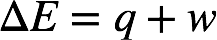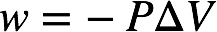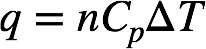# Problem: Consider a balloon filled with helium at the following conditions.313 g He 1.00 atm 1910. L Molar Heat Capacity = 20.8 J/°C • molThe temperature of this balloon is decreased by 41.6°C as the volume decreases to 1643 L, with the pressure remaining constant. Determine q, w, and ΔE (in kJ) for the compression of the balloon.

###### FREE Expert Solution

Recall: The change in internal energy (ΔE or ΔU) is given by:The formula for work at constant pressure is:where ΔV = change in volume (final V – initial V).

The formula for heat at constant pressure is:where n = moles of compound, Cp = molar heat capacity at constant pressure, and ΔT = change in temperature (final T – initial T).

86% (425 ratings)###### Problem Details

Consider a balloon filled with helium at the following conditions.

313 g He

1.00 atm

1910. L

Molar Heat Capacity = 20.8 J/°C • mol

The temperature of this balloon is decreased by 41.6°C as the volume decreases to 1643 L, with the pressure remaining constant. Determine q, w, and ΔE (in kJ) for the compression of the balloon.